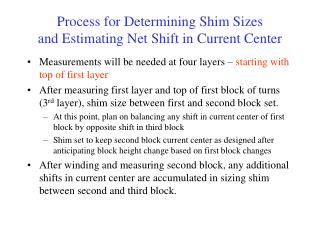# Process for Determining Shim Sizes and Estimating Net Shift in Current Center - PowerPoint PPT PresentationDownload PresentationProcess for Determining Shim Sizes and Estimating Net Shift in Current Center

Process for Determining Shim Sizes and Estimating Net Shift in Current Center
Download Presentation## Process for Determining Shim Sizes and Estimating Net Shift in Current Center

- - - - - - - - - - - - - - - - - - - - - - - - - - - E N D - - - - - - - - - - - - - - - - - - - - - - - - - - -
##### Presentation Transcript

1. Process for Determining Shim Sizesand Estimating Net Shift in Current Center • Measurements will be needed at four layers – starting with top of first layer • After measuring first layer and top of first block of turns (3rd layer), shim size between first and second block set. • At this point, plan on balancing any shift in current center of first block by opposite shift in third block • Shim set to keep second block current center as designed after anticipating block height change based on first block changes • After winding and measuring second block, any additional shifts in current center are accumulated in sizing shim between second and third block.

2. Ideal Winding aligns with 4 ProE Surfacesused with Romer Arm/Power Inspect Software Top of Third Block Top of Second Block Top of First Block Top of First Layer

3. Measure Base, Wind First Block, and Measure Top If Measurement of base of first block not possible measure top of first layer and estimate full block height by extrapolating Assumes ProE Surface of top of first layer available d2 d1x h1a = h1d + (d2-d1x)*3/2 x1a = x1d +0.25*d2+0.75*d1x Assuming (d2-d1)/3 = (d2-d1x)/2 d1 = d2 – (d2-d1x)*3/2 d2+d1 = 2*d2 – (d2-d1x)*3/2 (d2+d1)/2 = d2 -3/4*(d2-d1x) = d2*1/4 +d1x*3/4

4. Estimate Second Block Height Target Maintaining Original Current Centerto Size First Shim h2e = h1a *n2/n1 s1a = x2e – x1a –h1a/2 – h2e/2 chose shim size close to s1a call this s1ap adjust x2e = x2d + s1ap-s1a (h2e remains the same) x2ep = x2d (before choosing shim)

5. x3ep = x3d - (.25*d2+.75*d1) Preliminary Estimate of Third Block Height and Current Center to Correct First Block adjust estimate for different shim thickness x3ep = x3d –(.25*d2+.75*d1)–(s1ap-s1a)*n2/n3

6. Wind and Measure Second BlockCalculate Actual Height and Current Center Note: Measuring to original ProE geometry. With shim adjustment and estimated second block height, d3 is not expected to be zero. If actual height, h2a = h2e, d3 will equal (h2e-h2d)/2 +(s1ap-s1a) Define deviation from this expected value as d3p = d3 – (h2e-h2d)/2 -(s1ap-s1a) d3 h2a = h2e + d3p x2a = x2e + d3p/2

7. x3ep2 = x3ep - d3p/2*n2/n3 Estimate Third Block Height and Re-estimate Third Block Current Centerto Size Second Shim Third block height estimate extrapolated from first and second block heights h3e = h2a*(n3/n2)+ (h2a*(n3/n2)-h1a) s2a = x3e – x2a – h2a/2 – h3e/2 chose second shim size close to s2a call this s2ap adjust x3e = x3ep2 + s2ap-s2a (h3e remains the same)

8. h3a = h3e + d4p x3a = x3e + d4p/2 Wind and Measure Third BlockCalculate Actual Height and Current Center Note: Again, measuring to original ProE geometry. With shim adjustment and estimated second block height, d4 is not expected to be zero. If actual height, h3a = h3e, d4 will equal x3e + h3e/2 –x3d - h3d/2 Define deviation from this expected value as d4p = d4 – (x3e + h3e/2 –x3d - h3d/2)

9. Calculate Average/Net Shift of Half Bundle Current Center There is no capability to adjust for deviations in the third block. Net shift in current center is then the uncorrected error in the third block, weighted by the numbers of turns dx0 = (x3a - x3ep2)*n3/(n1+n2+n3)

10. Simplification • For the Twisted Racetrack Coil (and Type C Coils) with n1=n2=n3 the shim sizes can be expressed as an equivalent, simple linear combination of the design shim thickness and the measurements taken: • s1a = s1d + 0.75*d1x - 1.75*d2 • s1ap = closest shim size to s1a • s2a = s2d – 1.125*d1x + 0.125*d2 - 2.5*d3 + 0.5*(s1ap-s1a) • A similar expression can be obtained for the Type A & B, but the coefficients are TBD